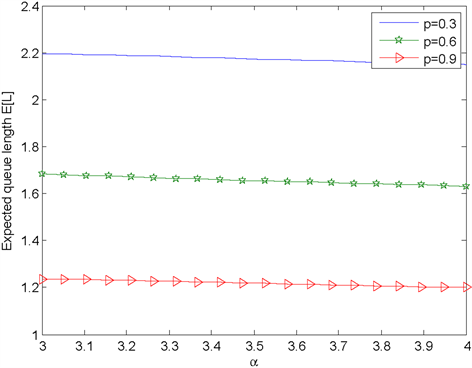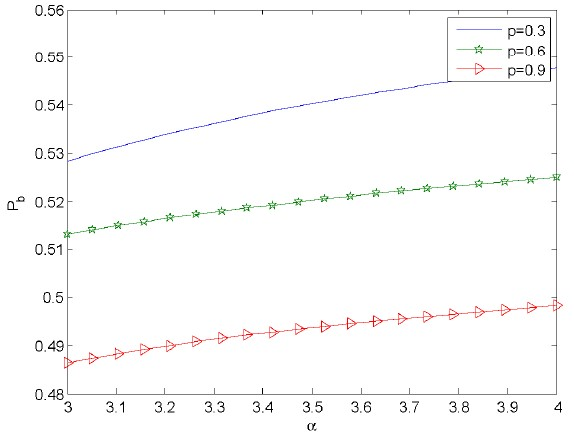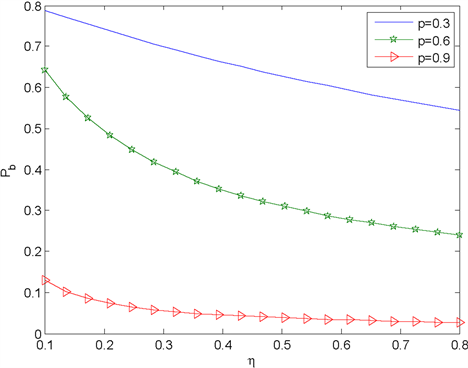﻿ 带有反馈的M/M/1重试工作休假排队模型 An M/M/1 Retrial Queue with Working Vacation and Feedback

Advances in Applied Mathematics
Vol. 08  No. 02 ( 2019 ), Article ID: 28841 , 9 pages
10.12677/AAM.2019.82024

An M/M/1 Retrial Queue with Working Vacation and Feedback

Juntong Li, Tao Li, Jinping Xu

School of Mathematics and Statistics, Shandong University of Technology, Zibo ShandongReceived: Jan. 22nd, 2019; accepted: Feb. 6th, 2019; published: Feb. 13th, 2019ABSTRACT

In this paper, we considered an M/M/1 retrial queue with working vacation and feedback. In order to obtain the necessary and sufficient condition for system to be stable, the matrix-analytic method is used. The stationary probability distribution and some performance measures were also derived. Then we give the conditional stochastic decomposition for the queue length in the orbit when the server is busy. Finally, the model parameters on the system's characteristics are presented by some numerical examples.

Keywords:Retrial, Working Vacation, Collision, Conditional Stochastic DecompositionCopyright © 2019 by authors and Hans Publishers Inc.1. 引言

2. 模型描述

1) 顾客的到达时间服从参数为 $\lambda$ 的泊松过程。

2) 正常服务期，服务员的服务速率服从参数为 $\mu$ 的指数分布，当重试组中没有顾客时，服务员开始一段时间为V的工作休假，且工作休假时间服从参数为 $\theta$ 的指数分布。在工作休假期间，服务员以低速率 $\eta$ 提供服务，且 $\eta <\mu$ 。当一个工作休假结束，若重试组中有顾客，则开始一个新的忙期，若重试组中没有顾客，则开始一个新的工作休假。

3) 当顾客到达系统发现服务员在忙，则顾客进入重试组等待，重试组中的顾客只有排在队列最前面的才能对服务器进行重试，直到重试成功并接受服务，重试时间服从参数为 $\alpha$ 的指数分布。

4) 顾客接受服务完毕后瞬间以概率 $p\left(0\le p\le 1\right)$ 离开系统，或以概率 $\stackrel{¯}{p}\left(\stackrel{¯}{p}=1-p\right)$ 返回重试组重新排队等待服务。

$Q\left(t\right)$ 表示t时刻重试组中的顾客数， $J\left(t\right)$ 表示t时刻服务员的状态，则服务员的四个状态如下：

$J\left(t\right)=\left\{\begin{array}{l}1,\text{\hspace{0.17em}}\text{\hspace{0.17em}}工作休假期,\text{\hspace{0.17em}}\text{\hspace{0.17em}}服务员忙\\ 2,\text{\hspace{0.17em}}\text{\hspace{0.17em}}工作休假期,\text{\hspace{0.17em}}\text{\hspace{0.17em}}服务员闲\\ 3,\text{\hspace{0.17em}}\text{\hspace{0.17em}}正常工作期,\text{\hspace{0.17em}}\text{\hspace{0.17em}}服务员忙\\ 4,\text{\hspace{0.17em}}\text{\hspace{0.17em}}正常工作期,\text{\hspace{0.17em}}\text{\hspace{0.17em}}服务员闲\end{array}$

$\stackrel{˜}{Q}=\left(\begin{array}{ccccc}{B}_{1}& {A}_{0}& & & \\ {A}_{2}& {A}_{1}& {A}_{0}& & \\ & {A}_{2}& {A}_{1}& {A}_{0}& \\ & & \ddots & \ddots & \ddots \end{array}\right)$

${B}_{1}=\left(\begin{array}{cccc}-\left(\lambda +\eta +\theta \right)& p\eta & \theta & 0\\ \lambda & -\lambda & 0& 0\\ 0& p\mu & -\left(\lambda +\mu \right)& 0\\ 0& 0& 0& 0\end{array}\right)$

${A}_{0}=\left(\begin{array}{cccc}\lambda & \stackrel{¯}{p}\eta & 0& 0\\ 0& 0& 0& 0\\ 0& 0& \lambda & \stackrel{¯}{p}\mu \\ 0& 0& 0& 0\end{array}\right)$

${A}_{2}=\left(\begin{array}{cccc}0& 0& 0& 0\\ \alpha & 0& 0& 0\\ 0& 0& 0& 0\\ 0& 0& \alpha & 0\end{array}\right)$

${A}_{1}=\left(\begin{array}{cccc}-\left(\lambda +\eta +\theta \right)& p\eta & \theta & 0\\ \lambda & -\left(\lambda +\alpha +\theta \right)& 0& \theta \\ 0& 0& -\left(\lambda +\mu \right)& p\mu \\ 0& 0& \lambda & -\left(\lambda +\alpha \right)\end{array}\right)$

3. 系统存在稳态的充要条件及稳态队长

$A={A}_{0}+{A}_{1}+{A}_{2}=\left(\begin{array}{cccc}-\left(\eta +\theta \right)& \eta & \theta & 0\\ \lambda +\alpha & -\left(\lambda +\alpha +\theta \right)& 0& \theta \\ 0& 0& -\mu & \mu \\ 0& 0& \lambda +\alpha & -\left(\lambda +\alpha \right)\end{array}\right)$

${\pi }^{\text{*}}\left(\begin{array}{cc}0& 0\\ \alpha & 0\end{array}\right)e>{\pi }^{\text{*}}\left(\begin{array}{cc}\lambda & \stackrel{¯}{p}\mu \\ 0& 0\end{array}\right)e$

$R=\left(\begin{array}{cccc}{r}_{1}& {r}_{2}& {r}_{3}& {r}_{4}\\ 0& 0& 0& 0\\ 0& 0& {r}_{5}& {r}_{6}\\ 0& 0& 0& 0\end{array}\right)$

${r}_{1}=\frac{\left[\left(\lambda +\eta +\theta \right)\left(\lambda +\alpha +\theta \right)-\stackrel{¯}{p}\eta \alpha -p\eta \lambda \right]-\sqrt{\Delta }}{2p\eta \alpha },$

${r}_{2}=\frac{\stackrel{¯}{p}\eta +{r}_{1}p\eta }{\lambda +\alpha +\theta },$

${r}_{3}=\frac{{r}_{1}{r}_{2}\alpha \theta +{r}_{2}\theta }{\left(\lambda +\mu \right)\left(\lambda +\alpha \right)-p\mu -p\mu \lambda -\left({r}_{6}\alpha -{r}_{1}\theta \right)\left(\lambda +\alpha \right)},$

${r}_{4}=\frac{{r}_{2}\theta +{r}_{3}p\mu }{\lambda +\alpha },$

${r}_{5}=\lambda \left(\lambda +\alpha +\stackrel{¯}{p}\mu \right),$

${r}_{6}=\lambda p\mu +\stackrel{¯}{p}p{\mu }^{2},$

$\Delta ={\left[\stackrel{¯}{p}\eta \alpha -\left(\lambda +\eta +\theta \right)\left(\lambda +\alpha +\theta \right)+p\eta \lambda \right]}^{2}-4p\eta \alpha \lambda \left(\stackrel{¯}{p}\eta +\lambda +\alpha +\theta \right).$

$\left\{\begin{array}{l}\left(\begin{array}{cc}0& 0\\ 0& 0\end{array}\right)={R}_{11}^{2}\left(\begin{array}{cc}0& 0\\ \alpha & 0\end{array}\right)+{R}_{11}\left(\begin{array}{cc}-\left(\lambda +\eta +\theta \right)& p\eta \\ \lambda & -\left(\lambda +\alpha +\theta \right)\end{array}\right)+\left(\begin{array}{cc}\lambda & \stackrel{¯}{p}\eta \\ 0& 0\end{array}\right),\\ \left(\begin{array}{cc}0& 0\\ 0& 0\end{array}\right)=\left({R}_{11}{R}_{12}+{R}_{12}{R}_{22}\right)\left(\begin{array}{cc}0& 0\\ \alpha & 0\end{array}\right)+{R}_{11}\left(\begin{array}{cc}\theta & 0\\ 0& \theta \end{array}\right)+{R}_{12}\left(\begin{array}{cc}-\left(\lambda +\mu \right)& p\mu \\ \lambda & -\left(\lambda +\alpha \right)\end{array}\right),\\ \left(\begin{array}{cc}0& 0\\ 0& 0\end{array}\right)={R}_{22}^{2}\left(\begin{array}{cc}0& 0\\ \alpha & 0\end{array}\right)+{R}_{22}\left(\begin{array}{cc}-\left(\lambda +\mu \right)& p\mu \\ \lambda & -\left(\lambda +\alpha \right)\end{array}\right)+\left(\begin{array}{cc}\lambda & \stackrel{¯}{p}\mu \\ 0& 0\end{array}\right).\end{array}$

${\pi }_{n}=\left({\pi }_{n1},{\pi }_{n2},{\pi }_{n3},{\pi }_{n4}\right),n\ge 0,$

${\pi }_{nj}=P\left\{Q=n,J=j\right\}=\underset{t\to \infty }{\mathrm{lim}}P\left\{Q\left(t\right)=n,J\left(t\right)=j\right\},\left(n,j\right)\in \Omega ,$

$\left\{\begin{array}{l}{\pi }_{n1}={\pi }_{01}{r}_{1}^{n},n\ge 1,\\ {\pi }_{n2}={\pi }_{01}{r}_{1}^{n-1}{r}_{2},n\ge 1,\\ {\pi }_{n3}={\pi }_{01}\left[\frac{{r}_{3}}{{r}_{5}-{r}_{1}}\left({r}_{5}^{n}-{r}_{1}^{n}\right)\right]+{\pi }_{03}{r}_{5}^{n},n\ge 1,\\ {\pi }_{n4}={\pi }_{01}\left[{r}_{4}{r}_{1}^{n-1}+\frac{{r}_{3}{r}_{6}}{{r}_{5}-{r}_{1}}\left({r}_{5}^{n-1}-{r}_{1}^{n-1}\right)\right]+{\pi }_{03}{r}_{5}^{n-1}{r}_{6},n\ge 1,\end{array}$ (1)

$\left\{\begin{array}{l}{\pi }_{02}=\frac{\left(\lambda +\eta +\theta \right)+{r}_{2}\alpha }{\lambda }{\pi }_{01},\\ {\pi }_{03}=\frac{{r}_{4}\alpha +\theta }{\left(\lambda +\mu \right)-{r}_{6}\alpha }{\pi }_{01}.\end{array}$ (2)

${\pi }_{n}=\left({\pi }_{n1},{\pi }_{n2},{\pi }_{n3},{\pi }_{n4}\right)={\pi }_{0}{R}^{n}=\left({\pi }_{01},{\pi }_{02},{\pi }_{03},{\pi }_{04}\right){R}^{n},n\ge 0$

${R}^{n}=\left(\begin{array}{cccc}{r}_{1}^{n}& {r}_{1}^{n-1}{r}_{2}& \frac{{r}_{3}}{{r}_{5}-{r}_{1}}\left({r}_{5}^{n}-{r}_{1}^{n}\right)& {r}_{4}{r}_{1}^{n-1}+\frac{{r}_{3}{r}_{6}}{{r}_{5}-{r}_{1}}\left({r}_{5}^{n-1}-{r}_{1}^{n-1}\right)\\ 0& 0& 0& 0\\ 0& 0& {r}_{5}^{n}& {r}_{5}^{n-1}{r}_{6}\\ 0& 0& 0& 0\end{array}\right),n\ge 1$

${R}^{n}$ 代入上述方程，我们可以得到(1)式。并且 ${\pi }_{0}$ 满足下述方程：

${\pi }_{0}\left({B}_{1}+R{A}_{2}\right)=0.$ (3)

$\underset{j=1}{\overset{4}{\sum }}\underset{n=0}{\overset{\infty }{\sum }}{\pi }_{nj}=1,$

$x=\frac{\left(1-{r}_{1}\right)\left(\lambda +\eta +\theta +{r}_{2}\alpha \right)+\left({r}_{1}+{r}_{2}+{r}_{4}\right)\lambda }{\lambda \left(1-{r}_{1}\right)},$

$y=\frac{\left({r}_{4}\alpha +\theta \right)\left(1-{r}_{5}+{r}_{5}{r}_{6}\right)}{\left(1-{r}_{5}\right)\left(\lambda +\mu -{r}_{6}\alpha \right)},$

$z=\frac{{r}_{3}\left({r}_{5}-{r}_{1}\right)+{r}_{3}{r}_{6}\left({r}_{1}+{r}_{5}\right)}{\left(1-{r}_{5}\right)\left(1-{r}_{1}\right)\left({r}_{5}-{r}_{1}\right)}.$

${P}_{1}=P\left\{J=1\right\}=\underset{n=0}{\overset{\infty }{\sum }}{\pi }_{n1}=\frac{1}{1-{r}_{1}}{\pi }_{01},$

${P}_{2}=P\left\{J=2\right\}=\underset{n=0}{\overset{\infty }{\sum }}{\pi }_{n2}=\frac{{r}_{2}}{\left(1-{r}_{1}\right){r}_{1}}{\pi }_{01},$

${P}_{3}=P\left\{J=3\right\}=\underset{n=0}{\overset{\infty }{\sum }}{\pi }_{n3}=\left[\frac{{r}_{3}}{\left(1-{r}_{5}\right)\left(1-{r}_{1}\right)}+\frac{{r}_{4}\alpha +\theta }{\left(1-{r}_{5}\right)\left[\left(\lambda +\mu \right)-{r}_{6}\alpha \right]}\right]{\pi }_{01},$

${P}_{4}=P\left\{J=4\right\}=\underset{n=1}{\overset{\infty }{\sum }}{\pi }_{n4}=\left\{\frac{{r}_{4}}{\left(1-{r}_{1}\right){r}_{1}}+\frac{{r}_{3}{r}_{6}\left({r}_{1}+{r}_{5}-1\right)}{\left(1-{r}_{1}\right)\left(1-{r}_{5}\right){r}_{1}{r}_{5}}+\frac{{r}_{6}\left({r}_{4}\alpha +\theta \right)}{\left(1-{r}_{5}\right)r\left[\left(\lambda +\mu \right)-{r}_{6}\right]}\right\}{\pi }_{01}.$

${P}_{b}=P\left\{J=1\right\}+P\left\{J=3\right\}={P}_{1}+{P}_{3}.$

${P}_{c}=P\left\{J=2\right\}+P\left\{J=4\right\}={P}_{2}+{P}_{4}=1-{P}_{b}.$

$\begin{array}{l}E\left[L\right]=\underset{n=1}{\overset{\infty }{\sum }}n\left({\pi }_{n1}+{\pi }_{n2}+{\pi }_{n3}+{\pi }_{n4}\right)\\ \text{\hspace{0.17em}}\text{\hspace{0.17em}}\text{\hspace{0.17em}}\text{\hspace{0.17em}}\text{\hspace{0.17em}}\text{\hspace{0.17em}}\text{ }\text{ }\text{ }\text{ }=\frac{\left({r}_{1}+{r}_{2}+{r}_{4}\right){\left(1-{r}_{5}\right)}^{2}+\left(1-{r}_{1}{r}_{5}\right){r}_{3}+\left(2-{r}_{1}-{r}_{5}\right){r}_{3}{r}_{6}}{{\left(1-{r}_{5}\right)}^{2}{\left(1-{r}_{1}\right)}^{2}}{\pi }_{01}+\frac{{r}_{5}+{r}_{6}}{{\left(1-{r}_{5}\right)}^{2}}{\pi }_{03}.\end{array}$

$\stackrel{˜}{L}$ 表示系统中的顾客数，我们可以得到

$E\left[\stackrel{˜}{L}\right]=\underset{n=1}{\overset{\infty }{\sum }}n\left({\pi }_{n2}+{\pi }_{n4}\right)+\underset{n=0}{\overset{\infty }{\sum }}\left(n+1\right)\left({\pi }_{n1}+{\pi }_{n3}\right)=E\left[L\right]+{P}_{1}+{P}_{3}=E\left[L\right]+{P}_{b}.$

4. 随机条件分解

${G}_{{Q}_{0}}\left(z\right)=\frac{1-{r}_{5}}{1-{r}_{5}z}.$

${Q}_{b}$ 表示本系统orbit中服务忙时的条件队长，如果 $\mu \alpha >\left(\lambda +\stackrel{¯}{p}\mu \right)\left(\lambda +\mu \right)$ ，我们可以得到

$\begin{array}{l}{G}_{{Q}_{b}}\left(z\right)=\underset{n=0}{\overset{\infty }{\sum }}P\left\{{Q}_{b}=n\right\}\cdot {z}^{n}=\underset{n=0}{\overset{\infty }{\sum }}\frac{{\pi }_{n1}+{\pi }_{n3}}{{P}_{b}}\cdot {z}^{n}\\ \text{\hspace{0.17em}}\text{\hspace{0.17em}}\text{\hspace{0.17em}}\text{\hspace{0.17em}}\text{\hspace{0.17em}}\text{\hspace{0.17em}}\text{\hspace{0.17em}}\text{\hspace{0.17em}}\text{\hspace{0.17em}}\text{\hspace{0.17em}}=\frac{1-{r}_{5}}{1-{r}_{5}z}\cdot \frac{\left[1-{r}_{5}z+{r}_{3}z+d\left(1-{r}_{1}z\right)\right]{\pi }_{01}}{\left(1-{r}_{1}z\right)\left(1-{r}_{5}\right){P}_{b}}={G}_{{Q}_{0}}\left(z\right){G}_{{Q}_{c}}\left(z\right)\end{array}$

5. 数值例子Figure 1. The expected queue length in the orbit with the change of $\alpha$Figure 2. The probability that the server is busy with the change of $\alpha$Figure 3. The expected queue length in the orbit with the change of $\eta$Figure 4. The probability that the server is busy with the change of $\eta$

6. 结论

An M/M/1 Retrial Queue with Working Vacation and Feedback[J]. 应用数学进展, 2019, 08(02): 210-218. https://doi.org/10.12677/AAM.2019.82024

1. 1. Tian, N. and Zhang, Z.G. (2006) Vacation Queueing Models-Theory and Applications. Springer Link, Berlin. https://doi.org/10.1007/978-0-387-33723-4

2. 2. Dragieva, V.I. (2011) Some Orbit Size Properties in the M/M/1//N Retrial Queue. International Conference on Queuing Theory & Network Applications. https://doi.org/10.1145/2021216.2021223

3. 3. Dragieva, V. and Phung-Duc, T. (2017) Two-Way Communication M/M/1//N Retrial Queue. In: Thomas, N. and Forshaw, M., Eds., Analytical and Stochastic Modelling Techniques and Applications (ASMTA 2017). Lecture Notes in Computer Science, Vol. 10378, Springer, Cham. https://doi.org/10.1007/978-3-319-61428-1_6

4. 4. Fedorova, E. and Voytikov, K. (2017) Retrial Queue M/G/1 with Impatient Calls under Heavy Load Condition. In: Dudin, A., Nazarov, A. and Kirpichnikov, A., Eds., Information Technologies and Mathematical Modelling. Queueing Theory and Applications (ITMM 2017). Communications in Computer and Information Science, Vol. 800, Springer, Cham. https://doi.org/10.1007/978-3-319-68069-9_28

5. 5. Servi, L.D. and Finn, S.G. (2002) M/M/1 Queue with Work-ing Vacations (M/M/1/WV). Performance Evaluation, 50, 41-52. https://doi.org/10.1016/S0166-5316(02)00057-3

6. 6. Do, T.V. (2010) M/M/1 Retrial Queue with Working Vaca-tions. Acta Informatica, 47, 67-75. https://doi.org/10.1007/s00236-009-0110-y

7. 7. Li, T., Zhang, L. and Gao, S. (2015) An M/M/1 Retrial Queue with Bernoulli-Schedule-Controlled Vacation and Vacation Interruption. ICIC Express Letters, 9, 3385-3392.

8. 8. Kumar, B.K., Madheswari, S.P. and Vijayakumar, A. (2002) The M/G/1 Retrial Queue with Feedback and Starting Failures. Applied Mathematical Modelling, 26, 1057-1075. https://doi.org/10.1016/S0307-904X(02)00061-6

9. 9. Madan, K.C. and Al-Rawwash, M. (2005) On the M/G/Lqueue with Feedback and Optional Server Vacations Based on a Single Vacation Policy. Applied Mathematics and Computation, 160, 909-919.

10. 10. Latouche, G. and Ramaswami, V. (1999) Introduction to Matrix Ananlytic Methods in Stochastic Modelling. ASA-SIAM Series on Applied Probability. https://doi.org/10.1137/1.9780898719734

11. 11. Li, T., Wang, Z. and Liu, Z. (2012) Geo/Geo/1 Retrial Queue with Working Vacations and Vacation Interruption. Journal of Applied Mathematics Computing, 39, 131-143. https://doi.org/10.1007/s12190-011-0516-x